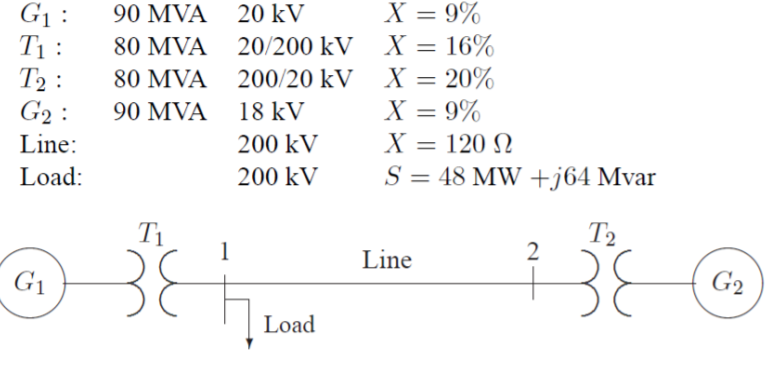# X = 9%X-16%X=20%X = 9%X=120 ΩS 48 MW +j64 Mvar1 90 MVA 20 kV80 MVA20/200kVT2: 80 MVA 200 20 kVG90 MVA 18 kVLine:Load200 kV200 kVΤιT2LineLoad

Question
391 views

Draw an impedance diagram for the electric power system shown in the figure attached, showing all impedances in per unit on a 100-MVA base. Choose 20kV as the voltage base for generator. The three-phase power and line-line ratings are given in the figure attached.help_outlineImage TranscriptioncloseX = 9% X-16% X=20% X = 9% X=120 Ω S 48 MW +j64 Mvar 1 90 MVA 20 kV 80 MVA 20/200kV T2: 80 MVA 200 20 kV G90 MVA 18 kV Line: Load 200 kV 200 kV Τι T2 Line Load fullscreen
check_circle

star
star
star
star
star
1 Rating
Step 1

We can obtain the impedance diagram by using below steps: -

The base voltage VBG1 on the LV side of T1 is 20 kV. Hence the base on its HV side is: -VB1 = 20 (200/20) = 200 kV

This fixes the base on the HV side of T2 at VB2 = 200 kV, and on its LV side atVBG2 = 200(20/200) = 20 kV

Step 2

The generator and transformer reactances in per unit on a 100 MVA base, as below: -

Step 3

The base impedance for the transmission line is

ZBL = (200)2/100 = 400 ohms

The per unit line reactance is

Line:    X = (120/400) = 0.30 per unit

The load impedance in ohms is:

ZL = (VL-L)2/(S*L(3-phase) = (200)2/(48-j6...

### Want to see the full answer?

See Solution

#### Want to see this answer and more?

Solutions are written by subject experts who are available 24/7. Questions are typically answered within 1 hour.*

See Solution
*Response times may vary by subject and question.
Tagged in

### Electrical Engineering